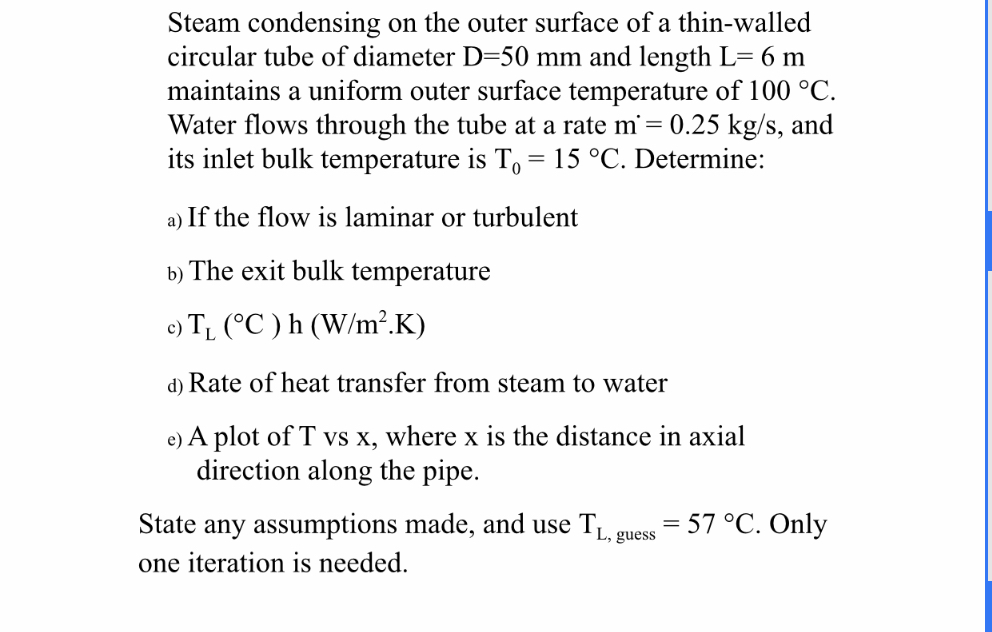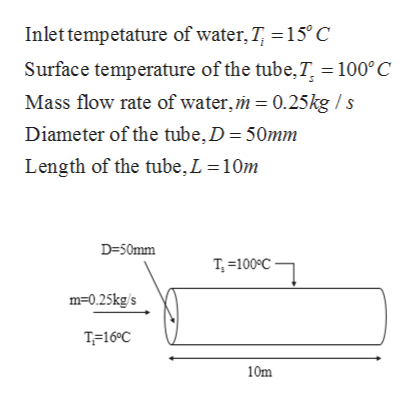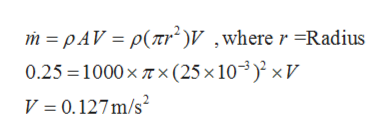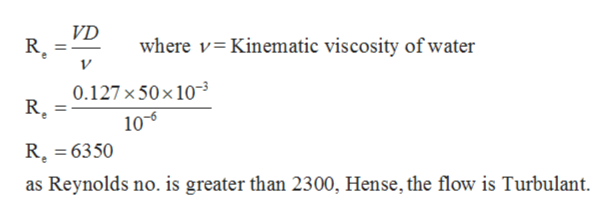Steam condensing on the outer surface of a thin-walledcircular tube of diameter D-50 mm and length L= 6 mmaintains a uniform outer surface temperature of 100 °CWater flows through the tube at a rate m= 0.25 kg/s, andits inlet bulk temperature is To= 15 °C. Determine:a) If the flow is laminar or turbulentb) The exit bulk temperaturee) T (C) h (W/m2.K)d) Rate of heat transfer from steam to watere) A plot of T vs x, where x is the distance in axialdirection along the pipeState any assumptions made, and use T57 °C. OnlyL, guessone iteration is needed.

Question

Please answer The test part d and e From this  problem The test is akredytacji solved thank youhelp_outlineImage TranscriptioncloseSteam condensing on the outer surface of a thin-walled circular tube of diameter D-50 mm and length L= 6 m maintains a uniform outer surface temperature of 100 °C Water flows through the tube at a rate m= 0.25 kg/s, and its inlet bulk temperature is To= 15 °C. Determine: a) If the flow is laminar or turbulent b) The exit bulk temperature e) T (C) h (W/m2.K) d) Rate of heat transfer from steam to water e) A plot of T vs x, where x is the distance in axial direction along the pipe State any assumptions made, and use T 57 °C. Only L, guess one iteration is needed. fullscreen
Step 1

Since we only answer up to 3 sub-parts, we’ll answer the first 3 parts. Please resubmit the question and specify the other subparts (up to 3) you’d like answered.

Given:help_outlineImage TranscriptioncloseInlet tempetature of water, T 15° C Surface temperature of the tube, T = 100°C Mass flow rate of water,m 0.25kg/s Diameter of the tube,D = 50mm Length of the tube,L =10m D-50mm T100C m-0.25kg/s T 16°C 10m fullscreen
Step 2

(a)

The velocity of water is calculated using the formula:help_outlineImage Transcriptionclosem pAV p(Tr2V ,where r -Radius 0.25 1000x Tx(25 x 103) xV V 0.127m/s fullscreen
Step 3

The Reynold no. is ...help_outlineImage TranscriptioncloseVD R where v Kinematic viscosity of water 0.127x50x10 10-5 R. 6350 as Reynolds no. is greater than 2300, Hense, the flow is Turbulant fullscreen

Want to see the full answer?

See Solution

Want to see this answer and more?

Our solutions are written by experts, many with advanced degrees, and available 24/7

See Solution
Tagged in

Heat Transfer